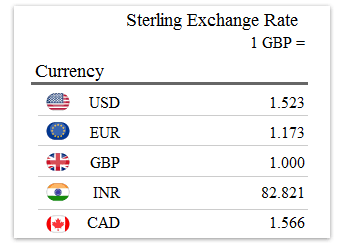## Classic Question 1: How many Canadian Dollars (CAD) can a trader buy with 400 Indian Rupees (IRP)?Step 1: Analyse the problem

We cannot convert directly from CAD to IRP, so we are going to have to use an intermediary currency. In this case, GBP. This technique is called cross multiplication/division.

Step 2: Cross multiply/divide

(400 ÷ 82.821) x 1.566

Ans = 7.56 CAD

So why did I do that combination of division and multiplication? Let’s look at the full process:

We need to convert 400 IRP into the base currency we have data for (GBP in this case). So 400 ÷ 82.821 = 4.8297 GBP. Then we need to know how many IRP our 4.8297 GBP is equivalent to. We can use the table again: 4.8297 x 1.566 = 7.56 CAD.

Comments are closed.